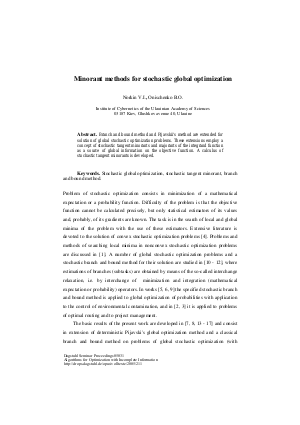Document# Minorant methods for stochastic global optimization

### Authors Vladimir Norkin, Boris. Onischenko## Files

DagSemProc.05031.14.pdf
• Filesize: 368 kB
• 9 pages

## Cite As

Vladimir Norkin and Boris. Onischenko. Minorant methods for stochastic global optimization. In Algorithms for Optimization with Incomplete Information. Dagstuhl Seminar Proceedings, Volume 5031, pp. 1-9, Schloss Dagstuhl - Leibniz-Zentrum für Informatik (2005)
https://doi.org/10.4230/DagSemProc.05031.14

## Abstract

We develop numerical methods for solution of stochastic global optimization problems: min\$[F(x)=Ef(x,Ã‚Â¦ÃƒËœ)| xin X]\$ and \$min[F(x)=P{f(x, Ã‚Â¦ÃƒËœ) Ã‚Â¡ÃƒÅ“0} | xin X]\$, where x is a finite dimensional decision vector with possible values in the set X, Ã‚Â¦ÃƒËœ is a random variable, \$f(x,Ã‚Â¦ÃƒËœ)\$ is a nonlinear function of variable x, E and P denote mathematical expectation and probability signs respectively. These methods are based on the concept of stochastic tangent minorant, which is a random function \$Ã‚Â¦Ãƒâ€¢(x,y, Ã‚Â¦ÃƒËœ)\$ of two variables x and y with expected value \$Ã‚Â¦Ã‚Âµ(x,y)=E Ã‚Â¦Ãƒâ€¢(x,y, Ã‚Â¦ÃƒËœ)\$ satisfying conditions: (i) \$Ã‚Â¦Ã‚Âµ(x,x)=F(x)\$, (ii) \$Ã‚Â¦Ã‚Âµ(x,y) Ã‚Â¡ÃƒÅ“F(x)\$ for all x,y. Tangent minorant is a source of information on a function global behavior. We develop a calculus of (stochastic) tangent minorants. We develop a stochastic analogue of PijavskiÃ‚Â¡Ã‚Â¯s global optimization method and a branch and bound method with stochastic minorant bounds. Applications to optimal facility location and network reliability optimization are discussed.
##### Keywords
• Stochastic global optimization
• stochastic tangent minorant
• branch and bound method

## Metrics

• Access Statistics
• Total Accesses (updated on a weekly basis)
0